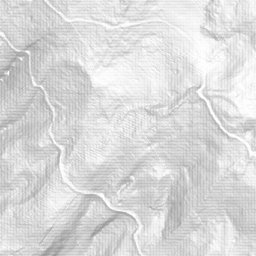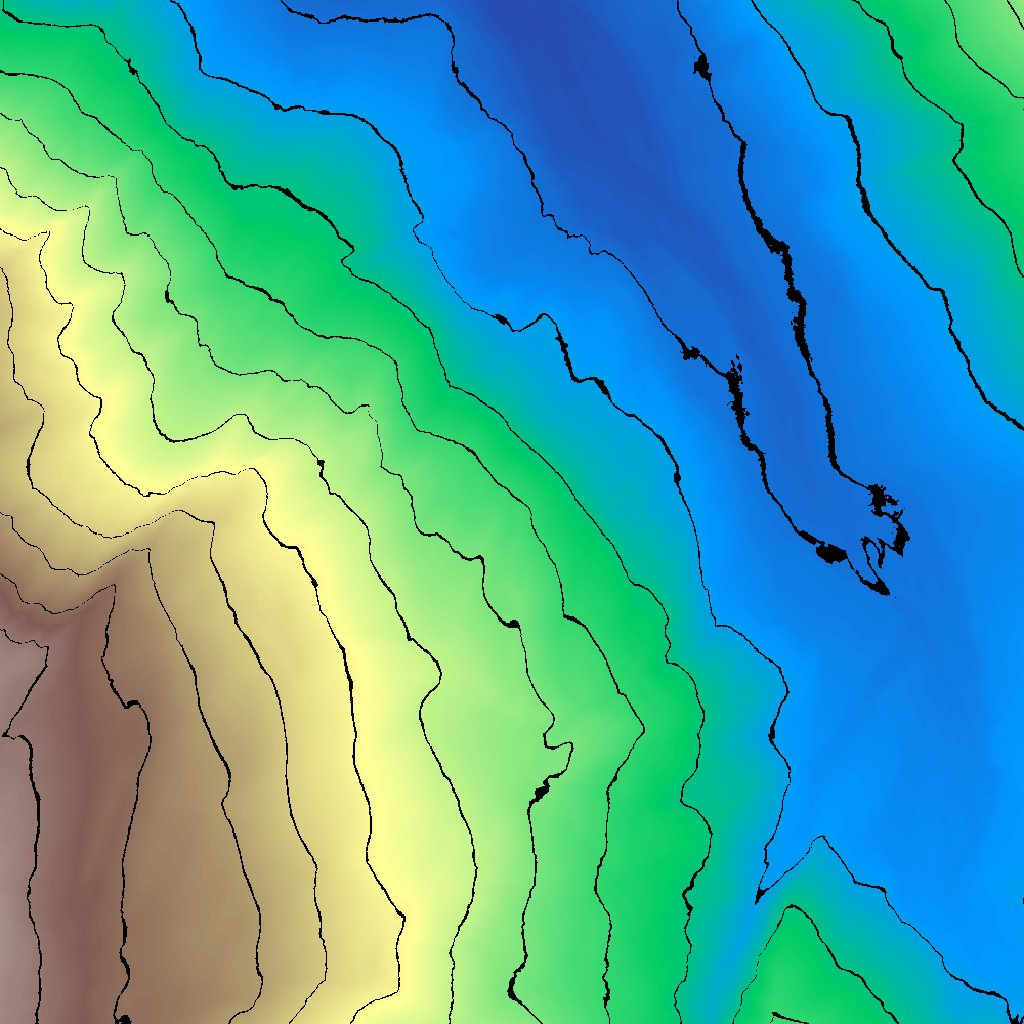# Custom Algorithm

Starting with `titiler>=0.8`, we added the possibility to apply custom algorithms on Image outputs from `tile`, `crop` or `preview` endpoints.

The algorithms are meant to overcome the limitation of `expression` (using numexpr) by allowing more complex operations.

We added a set of custom algorithms:

### Usage¶

``````# return a
httpx.get(
"http://127.0.0.1:8081/cog/tiles/16/34059/23335",
params={
"buffer": 3,  # By default hillshade will crop the output with a 3pixel buffer, so we need to apply a buffer on the tile
},
)
````````````# Pass algorithm parameter as a json string
httpx.get(
"http://127.0.0.1:8081/cog/preview",
params={
"algorithm": "contour",
"algorithm_params": json.dumps({"minz": 1600, "maxz": 2100}) # algorithm params HAVE TO be provided as a JSON string
},
)
``````A titiler'w `Algorithm` must be defined using `titiler.core.algorithm.BaseAlgorithm` base class.

``````class BaseAlgorithm(BaseModel, metaclass=abc.ABCMeta):
"""Algorithm baseclass.

Note: attribute starting with `input_` or `output_` are considered as metadata

"""

input_nbands: int
output_nbands: int
output_dtype: str
output_min: Optional[Sequence]
output_max: Optional[Sequence]

@abc.abstractmethod
def __call__(self, img: ImageData) -> ImageData:
"""Apply algorithm"""
...

class Config:
"""Config for model."""

extra = "allow"
``````

This base class defines that algorithm:

• HAVE TO implement an `__call__` method which takes an ImageData as input and return an ImageData. Using `__call__` let us use the object as a callable (e.g `Algorithm(**kwargs)(image)`).

• can have input/output metadata (informative)

• can have`parameters` (enabled by `extra = "allow"` pydantic config)

Here is a simple example of a custom Algorithm:

``````from titiler.core.algorithm import BaseAlgorithm
from rio_tiler.models import ImageData

class Multiply(BaseAlgorithm):

# Parameters
factor: int # There is no default, which means calls to this algorithm without any parameter will fail

# We don't set any metadata for this Algorithm

def __call__(self, img: ImageData) -> ImageData:
# Multiply image data bcy factor
data = img.data * self.factor

# Create output ImageData
return ImageData(
data,
assets=img.assets,
crs=img.crs,
bounds=img.bounds,
)
``````

#### Class Vs script¶

Using a Pydantic's `BaseModel` class to construct the custom algorithm enables two things parametrization and type casting/validation.

If we look at the `Multiply` algorithm, we can see it needs a `factor` parameter. In Titiler (in the post_process dependency) we will pass this parameter via query string (e.g `/preview.png?algo=multiply&algo_parameter={"factor":3}`) and pydantic will make sure we use the right types/values.

``````# Available algorithm
algo = {"multiply": Multiply}

def post_process_dependency(
algorithm: Literal[tuple(algo.keys())] = Query(None, description="Algorithm name"),
algorithm_params: str = Query(None, description="Algorithm parameter"),
) -> Optional[BaseAlgorithm]:
"""Data Post-Processing dependency."""
# Parse `algorithm_params` JSON parameters
kwargs = json.loads(algorithm_params) if algorithm_params else {}
if algorithm:
# Here we construct the Algorithm Object with the kwargs from the `algo_params` query-parameter
return algo[algorithm](**kwargs)

return None
``````

## Dependency¶

To be able to use your own algorithm in titiler's endpoint you need to create a `Dependency` to tell the application what algorithm are available.

To ease the dependency creation, we added a `dependency` property in the `titiler.core.algorithm.Algorithms` class, which will return a FastAPI dependency to be added to the endpoints.

Note: The `Algorithms` class is a store for the algorithm that can be extented using the `.register()` method.

``````from typing import Callable
from titiler.core.algorithm import algorithms as default_algorithms
from titiler.core.algorithm import Algorithms
from titiler.core.factory import TilerFactory

# Add the `Multiply` algorithm to the default ones
algorithms: Algorithms = default_algorithms.register({"multiply": Multiply})

# Create a PostProcessParams dependency
PostProcessParams: Callable = algorithms.dependency

endpoints = TilerFactory(process_dependency=PostProcessParams)
``````

### Order of operation¶

When creating a map tile (or other images), we will fist apply the `algorithm` then the `rescaling` and finally the `color_formula`.

``````with reader(url as src_dst:
image = src_dst.tile(
x,
y,
z,
)
dst_colormap = getattr(src_dst, "colormap", None)

# Apply algorithm
if post_process:
image = post_process(image)

# Apply data rescaling
if rescale:
image.rescale(rescale)

# Apply color-formula
if color_formula:
image.apply_color_formula(color_formula)

# Determine the format
if not format:
format = ImageType.jpeg if image.mask.all() else ImageType.png

# Image Rendering
return image.render(
img_format=format.driver,
colormap=colormap or dst_colormap,
**format.profile,
)
``````